# GMAT Math : Solving linear equations with two unknowns

## Example Questions

1 3 Next →

### Example Question #21 : Solving Linear Equations With Two Unknowns

Solve the following system of equations: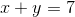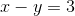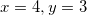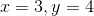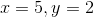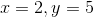Explanation:

To solve, I used the elimination method by adding the tqo equations together. That eliminates y and leaves you with: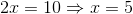Therefore,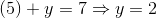### Example Question #22 : Solving Linear Equations With Two Unknowns

Which of the following equations is parallel to the line given by the equation: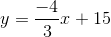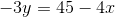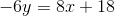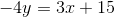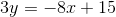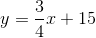Explanation:

For lines to be parallel, their equations must have equal slopes. Therefore, we are looking for another line with a slope of -4/3. If we convert the equation:into slope - intercept form, we get: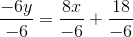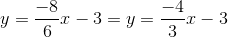It has the same slope, and therefore is parallel to the original line.

### Example Question #23 : Solving Linear Equations With Two Unknowns

The equations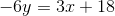and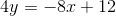intersect at the point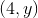. What is y?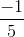Explanation:

The easiest way to solve the problem is to solve for y in one of the equations and then plug it into the other equation: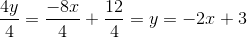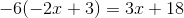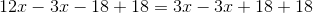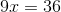We can then plug that x-value into one of the original equations: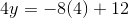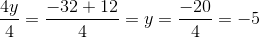1 3 Next →

Tired of practice problems?

Try live online GMAT prep today.